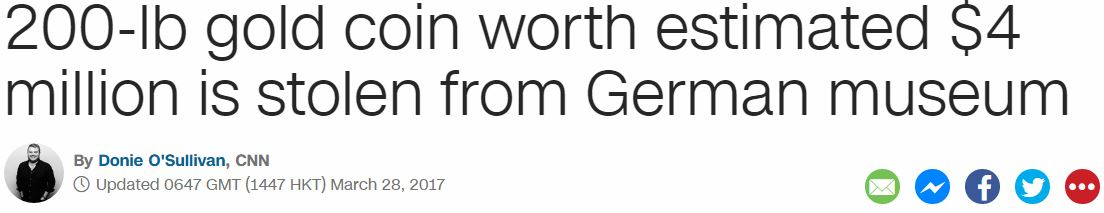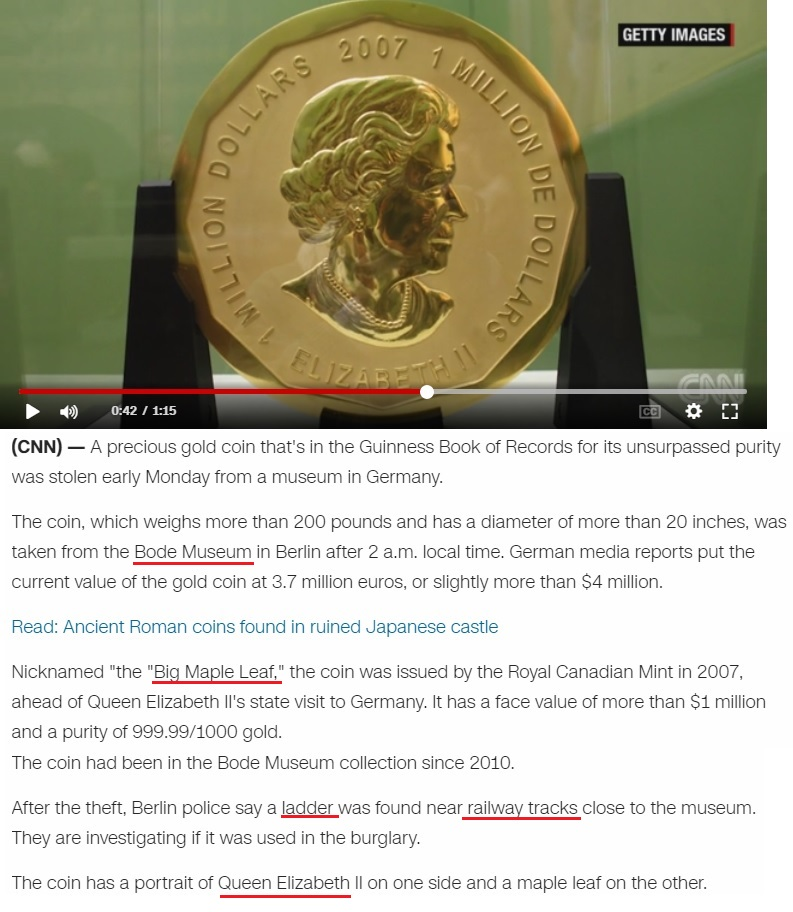## Cipher## Mar 28, 2017

### CNN - 200-lb gold coin stolen from German museum (not really)

This has to be the most hilarious story of 2017 so far.. Absolutely nothing about this makes sense so I'll just ignore all the idiocy and just focus on the numbers, also the coin is definitely not stolen, this whole story is a joke... http://edition.cnn.com/2017/03/27/europe/german-coin-stolen-trnd/index.htmlThis is coming on 87th day of the year - Twenty eighth of March = 7+4+4+4+7+2 + 4+9+2+1+7+1 + 3+3 + 5+8+9+6+1 = 87 (Reverse Reduced)
"Queen Elizabeth II" = 8+3+5+5+5 + 5+3+9+8+1+2+5+2+8 + 9+9 = 87 (Reduced)

Twenty eighth of March = 20+23+5+14+20+25 + 5+9+7+8+20+8 + 15+6 + 13+1+18+3+8 = 228 (Ordinal)
Queen Elizabeth = 10+6+22+22+13 + 22+15+18+1+26+25+22+7+19 = 228 (Reverse Ordinal
golden coin = 7+50+20+4+5+40 + 3+50+9+40 = 228 (Jewish)

The coin is nicknamed "Big Maple Leaf" = 2+9+7 + 4+1+7+3+5 + 3+5+1+6 = 53 (Reduced)
"golden coin" = 7+6+3+4+5+5 + 3+6+9+5 = 53 (Reduced)
It was sitting in "Bode Museum" = 7+3+5+4 + 5+6+8+4+6+5 = 53 (Reverse Reduced)
The coin has a face value of "one million" = 3+4+4 + 5+9+6+6+9+3+4 = 53 (Reverse Reduced)It's very important to note that coin is 53 centimeters in diameter - "Fifty three centimeters" = 21+18+21+7+2 + 7+19+9+22+22 + 24+22+13+7+18+14+22+7+22+9+8 = 314 (Reverse Ordinal)The ladder was supposedly found near "railway tracks" = 9+1+9+3+5+1+7 + 2+9+1+3+2+1 = 53 (Reduced)

Bode Museum is located in Berlin, Germany - https://en.wikipedia.org/wiki/Bode_MuseumThis is coming on 28th day of the month (33 and 28 are the only 2 numbers that sum to 156 in the first 300)
"Twenty eight" = 20+23+5+14+20+25 + 5+9+7+8+20 = 156 (Ordinal)

German Museum = 2+4+9+5+8+4 + 5+6+8+4+6+5 = 66 (Reverse Reduced)
Queen Elizabeth = 1+6+4+4+4 + 4+6+9+1+8+7+4+7+1 = 66 (Reverse
On Wikipedia they call it a  "solid gold coin" = 1+6+3+9+4 + 7+6+3+4 + 3+6+9+5 = 66 (Reduced)
This is happening 24 days before Elizabeth's birthday which is also 3 weeks and 3 days (note that coin is 24-carat gold)

This happened on a fitting date numerology

3/28/2017 = 3+2+8+2+0+1+7 = 23 - "Coin" = 3+6+9+5 = 23 (Reduced)
3/28/2017 = 3+28+2+0+1+7 = 41 - "Burglary" = 2+3+9+7+3+1+9+7 = 41 (Reduced), "Coin" = 3+15+9+14 = 41 (Ordinal)

There are also some 118's popping up
"Ladder" = 15+26+23+23+22+9 = 118 (Reverse Ordinal)
"fifty three centimeters" = 6+9+6+2+7 + 2+8+9+5+5 + 3+5+5+2+9+4+5+2+5+9+10 = 118 (S Exception)
"one million" = 15+14+5 + 13+9+12+12+9+15+14 = 118 (Ordinal)
"Bode Museum" = 2+15+4+5 + 13+21+19+5+21+13 = 118 (Ordinal)
Bode Museum was reopened on 10/18/2006